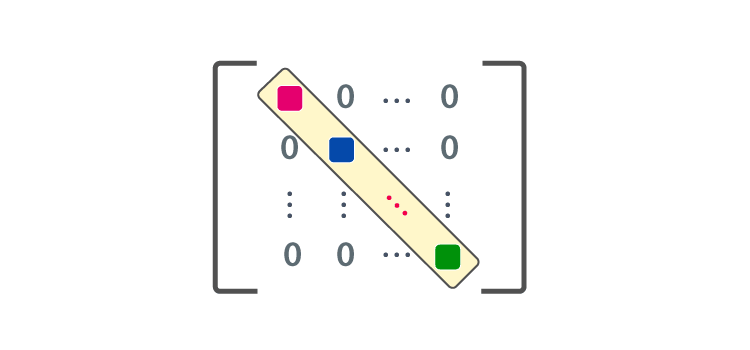Jet Set Go! All about Aeroplanes Jet Set Go! All about Aeroplanes

# Diagonal Matrix

A square matrix in which every element except the principal diagonal elements is zero is called a Diagonal Matrix. A square matrix D = [dij]n x n will be called a diagonal matrix if dij = 0, whenever i is not equal to j. There are many types of matrices like the Identity matrix.## Properties of Diagonal Matrix

Let’s learn about the properties of the diagonal matrix now.

Property 1: Same order diagonal matrices gives a diagonal matrix only after addition or multiplication.

Example:

$$\begin{array}{l}If\ P =\begin{bmatrix} 2 & 0\\ 0 & 4 \end{bmatrix}, and\end{array}$$
$$\begin{array}{l}Q = \begin{bmatrix} 4 & 0\\ 0 & 3 \end{bmatrix}\end{array}$$
$$\begin{array}{l}P + Q = \begin{bmatrix} 2 & 0\\ 0 & 4 \end{bmatrix} + \begin{bmatrix} 4 & 0\\ 0 & 3 \end{bmatrix}\end{array}$$
$$\begin{array}{l}P + Q = \begin{bmatrix} 2 + 4 & 0 + 0 \\ 0+0 & 4 + 3\end{bmatrix}\end{array}$$
$$\begin{array}{l}P + Q = \begin{bmatrix} 6 & 0\\ 0 & 7 \end{bmatrix}\end{array}$$

Property 2: Transpose of the diagonal matrix D is as the same matrix. D = DT

$$\begin{array}{l}\text{If p = }\begin{bmatrix} 2 & 0\\ 0 & 4 \end{bmatrix} \text{ then, } P^T= \begin{bmatrix} 2 & 0\\ 0 & 4 \end{bmatrix}\end{array}$$

Property 3: Under Multiplication, Diagonal Matrices are commutative, i. e. PQ = QP

$$\begin{array}{l}\text{ If P} = \begin{bmatrix} 2 & 0\\ 0 & 4 \end{bmatrix} \text{ and } Q = \begin{bmatrix} 4 & 0\\ 0 & 3 \end{bmatrix}\end{array}$$
$$\begin{array}{l}P \times Q =\begin{bmatrix} 8+0 & 0 + 0 \\ 0 + 0 & 12+0 \end{bmatrix}\end{array}$$
$$\begin{array}{l}\begin{bmatrix} 8 & 0\\ 0 & 12 \end{bmatrix}\end{array}$$
$$\begin{array}{l}Q \times P = \begin{bmatrix} 8+0 & 0 + 0 \\0 + 0& 12+0 \end{bmatrix}\end{array}$$
$$\begin{array}{l}\begin{bmatrix} 8 & 0\\ 0 & 12 \end{bmatrix}\end{array}$$

PQ = QP

### What is Block Diagonal Matrix?

A matrix which is split into blocks is called a block matrix. In such type of square matrix, off-diagonal blocks are zero matrices and main diagonal blocks square matrices. Here, the non-diagonal blocks are zero. Dij = 0 when i is not equal to j, then D is called a block diagonal matrix.

n x n order block diagonal matrix

$$\begin{array}{l}\begin{bmatrix} a_{11} & 0 & 0 & . & . & 0\\ 0 & a_{22} & 0 & .& . &0 \\ 0& 0 & a_{33} & .& . & 0\\ .& . & . &. & .&. \\ .& . & . & . & . &. \\ 0& 0 & 0 &0 &0 & a_{nn} \end{bmatrix}\end{array}$$

### Inverse of a Diagonal Matrix

If the elements on the main diagonal are the inverse of the corresponding element on the main diagonal of the D, then D is a diagonal matrix.

$$\begin{array}{l}Let\ D = \begin{bmatrix} a_{11} & 0& 0\\ 0 & a_{22} & 0\\ 0& 0 & a_{33} \end{bmatrix}\end{array}$$

Determinants of the above matrix:

$$\begin{array}{l}\left | D \right | = a_{11}\begin{bmatrix} a_{22} & 0\\ 0 & a_{33} \end{bmatrix}+ 0 \begin{bmatrix} 0& 0\\ 0 & a_{33} \end{bmatrix} + 0 \begin{bmatrix} 0 & a_{22} & \\ 0 & 0 \end{bmatrix}\end{array}$$

= a11a22a33

$$\begin{array}{l}Adj D = \begin{bmatrix} a_{22}a_{33} & 0& 0\\ 0 & a_{11}a_{33} & 0\\ 0& 0 & a_{11}a_{22} \end{bmatrix}\end{array}$$
$$\begin{array}{l}D^{-1} = \frac{1}{\left | D \right |} adj D\end{array}$$

=

$$\begin{array}{l}\frac{1}{a_{11}a_{22}a_{33}} \begin{bmatrix} a_{22}a_{33} & 0& 0\\ 0 & a_{11}a_{33} & 0\\ 0& 0 & a_{11}a_{22} \end{bmatrix}\end{array}$$
$$\begin{array}{l}\begin{bmatrix} \frac{1}{a_{11}} &0 & 0\\ 0 & \frac{1}{a_{22}} &0 \\ 0& 0 & \frac{1}{a_{33}} \end{bmatrix}\end{array}$$

### Anti Diagonal Matrix

If the entries in the matrix are all zero except the ones on the diagonals from lower left corner to the other upper side(right) corner are not zero, it is anti diagonal matrix.

It is of the form:

$$\begin{array}{l}\begin{bmatrix} 0 & 0 &a_{13} \\ 0 & a_{22}&0 \\ a_{31}& 0 & 0 \end{bmatrix}\end{array}$$

Register at BYJU’S to study many more interesting mathematical topics and concepts.